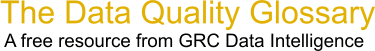Choose Index below for a list of all words and phrases defined in this glossary.

# Standard Deviation

index | Index

## Standard deviation - definitions

Standard deviation (Ïƒ or s) : A widely used measure of variability that expresses the measure of spread in a set of items. The standard deviation is a value such that approximately 68 percent of the items in a set fall within a range of the mean plus or minus the standard deviation. For data from a large sample of a population of items, the standard deviation Ïƒ (standard deviation of a population) or s (standard deviation of a sample) is expressed as :

```   s (Ïƒ ) = standard deviation of a sample (population)
d = the deviation of any item from the mean or average
n = the number of items in the sample
Ïƒ = "the sum of".
```

[Category=Data Quality ]

Source: Larry English, http://www.iaidq.com/main/glossary.shtml, 10-Feb-2009 12:48

These advertisers support this free service

Standard deviation - The standard deviation is a measure of variation that describes the spread around the mean for a set of data values.

[Category=Database Marketing ]

Source: NextMark, 19 August 2009 16:18:53, https://www.nextmark.com/resources/glossary-of-terms/ ?glossaryTermId=a0800000000FVTOAA4Standard deviation (statistical) - A computed measure of vari- ability indicating the spread of the data set around the mean.

[Category=Quality ]

Source: American Society for Quality, 23 October 2010 09:02:22, http://www.asq.org/glossary/Standard Deviation - A measure of the dispersion or imprecision of a sample or population distribution expressed as the positive square root of the variance and has the same unit of measurement as the mean.

[Category=Quality Assurance ]

Source: Massachusetts Department of Environmental Protection, 28 March 2011 09:05:03, http://www.mass.gov/dep/cleanup/laws/glossa01.htmstandard deviation - [statistics] A statistical measure of the spread of values from their mean, calculated as the square root of the sum of the squared deviations from the mean value, divided by the number of elements minus one. The standard deviation for a distribution is the square root of the variance.

[Category=Geospatial ]

Source: esri, 29 August 2012 08:16:06, http://support.esri.com/en/knowledgebase/GISDictionary/term/abbreviationData Quality Glossary.  A free resource from GRC Data Intelligence. For comments, questions or feedback: dqglossary@grcdi.nl